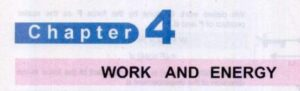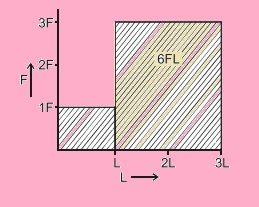Hello, students welcome to the new post. In this post, we will discuss Fsc 1st year physics chapter 4 solved exercise. We will learn all exercise questions of Fsc part one physics chapter 4. All questions will be discussed with the details. In the previous tutorial, we discussed chapter 3 exercise short question. So let get started

### Fsc 1st year physics chapter 4 solved exercise

Q 4.1:  Solution

• In these two conditions, there is no work done since the value of displacement for both objects or bodies is zero.
• As we know
• w= F.d
• =Fdcosθ
• as the value of displacement is zero so
• W=F(0)Cosθ
• so Work done is zero

Q 4.2:  Solution

• As we given
• m=10 kg
• h= 10 m
• He has to find work done in kilojoules
• Work done =w=mgh
• putting values
• W= 10 x 10x 9.8
• W=980 J
• W=(980 x1000)/1000
• 1000= 1 Kilo
• 980/1000 kJ

Q 4.3:  Solution

• To understand this problem diagram is created here you can see.
• Due to Force F distance L is covered so the work done is FL Accordioning to our condition distance is 2L due to force of 3F. In results
• W= 3F.2L
• 6FL
• So total work done will be
• W=Fl+ 6FL= 7FLQ 4.4:  Solution

• For the first condition
• m=50 kg
• h=50 cm=0.5m
• Value of work for this condition
• Wx= mgh
• =50 x0.5 x 9.8
• =245 J
• For the second condition
• m=50kg
• d=2m
• F=50 N
• The value of work for this condition is
• Wy=F.d
• 50 x 2
• =100 J
• More work done in the first condition

Q 4.5:  Solution

• As we know one Joule = 1 N x 1 m
• If one N force is applied an object to move it one-meter height. In the results work done stored in the object in form of P.E that is one-joule body has the ability to perform one-joule work

Q 4.6:  Solution

• The ball is at a height of h1 from the table and the table at h2 height from the ground. According to ground height of the ball is h1+h2.
• Potential energy stored in the ball is mgh1 and mg(h1+h2). Both students are correct one is taking the table as the first point and other ground.Q 4.7  Solution

• When the rocket re-enters into the atmosphere then its some K.E uses against the air friction and agins dust particles that exist in form of heat that causes turn nose of the racket hot

Q 4.8  Solution

• a: Compressed spring has elastic P.E
• b: Water in high dam has gravitational p.E
• c: The moving car has K.E

Q 4.8  Solution

• For curtain, height cup has gravitational P.E. When the cup drops down its gravitational P.E reduces, and K.E rises. Before colliding to the grounds its K.E has extreme value and after colliding converting to sound energy. With that heat energy and in energy that is used to break the cup in case of air friction is not counted.
• If friction is exist then some portion of K.E is used to reduce the friction value.

Q 4.9  Solution

• The elastic potential energy stored in the catapult is transferred to the stone in form of K.E
• When stone collides to the window its K.E is converted into sound, heat and energy used for breaking the window.

That is all about the Fsc 1st year physics chapter 4 solved exercise. I have explained all questions of chapter 4. I hope that will helps you to understand and resolve your queries. In next post, we will discuss the chapter 5 questions till than have a nice day.